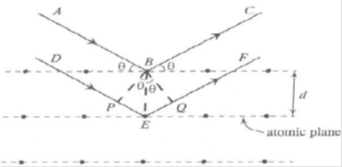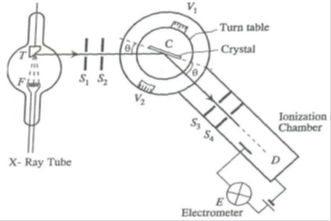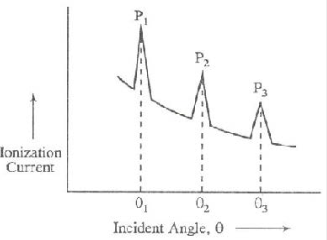Bragg’s spectrometer: X-rays of wavelength ‘λ’ be incident upon the crystal at an angle ‘θ’. The crystal acts as a series of parallel reflecting planes. The intensity of the reflected beam at certain angles will be maximum when the path difference between the two reflected waves from two different planes is nλ. Lattice planes are separated by a distance ‘d’. From figurePath difference = PE EQ = BE sinθ BE sinθ = d sinθ d sinθ = 2d sinθ

Intensity of the reflected light will be maximum when path difference is nλ.

i.e., 2d sinθ = nλ

This result is known as Bragg’s law.

Where ‘θ’ is called Bragg angle or glancing angle and ‘n’ is the order of diffraction.

Bragg’s X-ray spectrometer:

• A source of X-rays (X-ray tube)
• A crystal held on a circular turn table provided with vernier
• A detector (ionization chamber)

X-rays from an X-ray tube collimated by two narrow slits S1 and S2 are allowed to fall upon the crystal C. The crystal is mounted on the turn table, which can rotate about a vertical axis and its position can be determined by vernier  V1. The table is provided with a radial arm which carries ionization chamber. This arm can also be rotated about the same vertical axis as the crystal. The position of this arm can be determined by the vernier  V2. The ionization chamber is connected to an electrometer E to measure the ionization current. The slits S3 and S4 limit the width of the diffracted beam.

The crystal table and ionization chamber are connected in such a way that the chamber turns through 2θ, when the crystal turns through θ.This graph is called X-ray spectrum. The peaks in the graph occur whenever Bragg’s law is satisfied. One can measure the interplanar spacing ‘d’ through Bragg’s relation 2d sinθ = nλ, by using the measured value of ‘θ’, the order ‘n’ and x-ray wavelength ‘λ’.